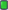Thread: CIU V2 Designer Preview View Single Post
#11Cesar82Registered User Join Date: May 2011 Location: Brazil Posts: 799 Thanks: 1,309 Thanked 1,702 Times in 580 PostsQuote:
 Originally Posted by GuptaI still didn't get why you used sqrt and other function
It is used to calculate and create a square Box with the flags if FlagByLine key (in setup.ini) is empty or does not exist.
Code:
```  if GetArrayLength(Result) = 0 then begin {If no results were obtained, it calculates the quantity to creates a square with the number of flags in the project.}
FL := Trunc(Sqrt(LN)) + (Trunc(Sqrt(LN)) mod 2);
SetArrayLength(Result, Trunc(Sqrt(LN)) + 1);
for I := 0 to GetArrayLength(Result) - 1 do begin
if FC + FL > LN then
FL := LN - FC;
Result[I] := FL;
FC := FC + FL
end;
end;```
This part adds only the numeral characters from temporary variable S2 to S3.
Code:
```    S3 := '';
for I := 1 to Length(S2) do
if StrToIntDef(S2[I], 10) < 10 then
S3 := S3 + S2[I];```
This part avoids including a line with zero flags at the top (LangBox Top).
Code:
```    if (S3 <> '') and (LN > FC)
and ((StrToIntDef(S3, 0) > 0) or (GetArrayLength(Result) > 0)) then
begin```
If the flags of the lines informed in FlagsByLine remain, this part creates new lines with the same number of flags as the previous line.
Code:
```  while (LN - FC > 0) and (FL > 0) do begin
if FC + FL > LN then
FL := LN - FC;
SetArrayLength(Result, GetArrayLength(Result) + 1);
Result[GetArrayLength(Result) - 1] := FL;
FC := FC + FL
end;```
This part creates a new line with the remaining flags at the end.
It also removes an empty line of flags from the end of the box for the correct CENTER alignment.
Code:
```  if GetArrayLength(Result) > 0 then begin
if LN - FC > 0 then
Result[GetArrayLength(Result) - 1] := FL + LN - FC;
if Result[GetArrayLength(Result) - 1] = 0 then
SetArrayLength(Result, GetArrayLength(Result) - 1);
end;```
See the example script I attached in the previous post.
Just change the values within GetValStr and SetArrayLength(Languages, 16).